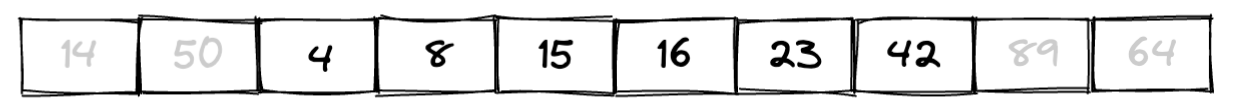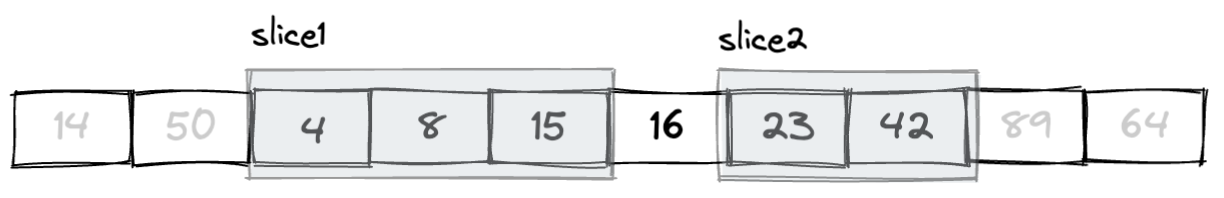# [為你自己學 Rust] 切片（Slice）``````let lost_numbers = [4, 8, 15, 16, 23, 42];
let slice1 = &lost_numbers[0..3];
let slice2 = &lost_numbers[4..6];

println!("{:?}", slice1);  // 印出 [4, 8, 15]
println!("{:?}", slice2);  // 印出 [23, 42]
``````

Slice 可以從字串、陣列或 Vector 之類的資料型態「切」一小塊出來，它一樣也有 `.len()` 可以用，但就沒有 `.capacity()` 了，因為它就只是個參照而已，它沒有什麼容量的問題。

### 省略索引值

``````let lost_numbers = vec![4, 8, 15, 16, 23, 42];
let first_two_nums = &lost_numbers[..2];  // 前 2 個
let last_three_nums = &lost_numbers[lost_numbers.len() - 3..];  // 後 3 個

println!("{:?}", first_two_nums); // 印出 [4, 8]
println!("{:?}", last_three_nums); // 印出 [16, 23, 42]
``````

``````let numbers = &lost_numbers[..];
``````

## 切片可以修改嗎？

``````let mut lost_numbers = vec![4, 8, 15, 16, 23, 42];
let nums = &lost_numbers[0..3];

nums = 123;
``````

``````let mut lost_numbers = vec![4, 8, 15, 16, 23, 42];
let nums = &mut lost_numbers[0..3];

nums = 5566;
println!("{:?}", lost_numbers); // 印出 [5566, 8, 15, 16, 23, 42]
``````

## 字串不是字串？

``````fn main() {
let book = "為你自己學 Rust";
publish_book(book);
}

fn publish_book(book: String) {
println!("{:?} 要上市囉！", book)
}
``````

`publish_book()` 函數會接一個 `String` 型別的參數，然後就把收到的參數印出來。這裡我用雙引號做了一個字串，然後傳進去，看起來沒什麼問題，但執行之後 Rust 又很不厭其煩的給你錯誤訊息：

``````\$ cargo run
error[E0308]: mismatched types
|
3 |     publish_book(book);
|     ------------ ^^^^- help: try using a conversion method: `.to_string()`
|     |            |
|     |            expected `String`, found `&str`
|     arguments to this function are incorrect
``````

``````fn publish_book(book: &str) {
println!("{:?} 要上市囉！", book)
}
``````

## 切片的型別？

Slice 就只是一個參照，它參照到某一段連續的資料，所以切片的型別就是它所參照到的資料的型別。我們先來看看底下這段範例：

``````let scores = [88, 12, 39, 15, 10, 28, 92];
let group1 = &scores[0..2]; // [88, 12]
let group2 = &scores[2..];  // [39, 15, 10, 28, 92]

println!("{}", calc_score(group1));
println!("{}", calc_score(group2));
``````

``````fn calc_score(scores: &[u16]) -> u16 {
scores.iter().sum()
}
``````

`&[u16]` 表示傳進來的參數是個陣列型的切片，前面的 `&` 表示它是一種參照型別，裡面的 `u16` 則是表示陣列裡的型別。知道這概念之後，上個段落提到的 `publish_book(book: &str)` 應該也就能看的懂了。

``````let book: String = String::from("為你自己學 Rust");
println!("{}", book);
``````

``````fn from(s: &str) -> String {
s.to_owned()
}
``````